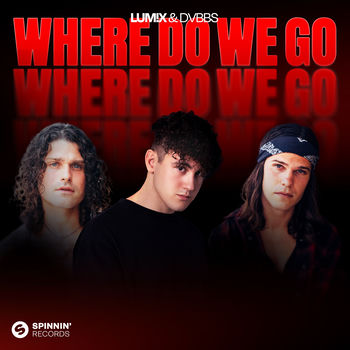Where Do We Go

# Where Do We Go

## Lyrics

Where do we go? Where do we go?

Maybe you're scared, up in the air

I know a place where we can go

Where do we hide? Where do we cry?

I know you're not the only one

Whenever you're down

I know a place where we belong

You feel like you're falling

You feel like you're far away

Lost in the dark, breaking apart

But I know you'll be safe

But there's no need to run

At the end of the day

I know a place, a place where we belong

Where do we go? Where do we go?

Maybe you're scared, up in the air

I know a place where we can go

Where do we hide? Where do we cry?

I know you're not the only one

Whenever you're down, down on the ground

I know a place where we belong

Where do we go? Where do we go?

Maybe you're scared, up in the air

I know a place where we can go

Where do we hide? Where do we cry?

I know you're not the only one

Whenever you're down, down on the ground

I know a place where we belong

(I know a place where we belong)

(Yeah, I know a place where we belong)

How can you sleep? How can you dream?

When you lost in the night

I'll lead the way, keeping you safe

You won't be left behind

Hold on to me tight, I'll ease your mind

You are never alone

'Cause I know a place where we belong

You feel like you're falling

You feel like you're far away

Lost in the dark, breaking apart

But I know you'll be safe

But there's no need to run

At the end of the day

I know a place, a place where we belong

Where do we go? Where do we go?

Maybe you're scared, up in the air

I know a place where we can go

Where do we hide? Where do we cry?

I know you're not the only one

Whenever you're down, down on the ground

I know a place where we belong

Where do we go? Where do we go?

Maybe you're scared, up in the air

I know a place where we can go

Where do we hide? Where do we cry?

I know you're not the only one

Whenever you're down, down on the ground

I know a place where we belong

by LUM!X, DVBBS